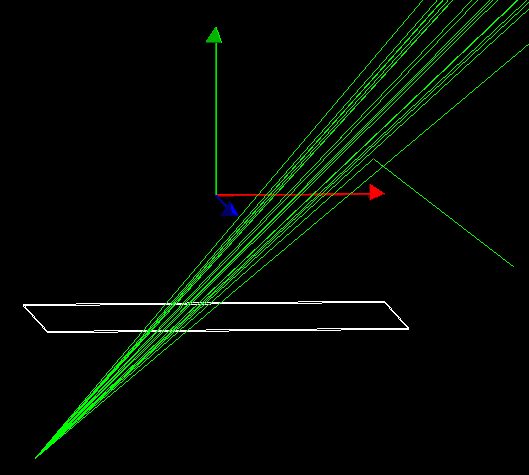Hi,
I want to specify the beam angle at about 70 ° like this:

But I don’t know how. So far, my code looks like:

``````G4double focus_size = 0.005*mm;
G4double x0 =  focus_size * (G4UniformRand()+4.0);
G4double y0 =  focus_size * (G4UniformRand()+4.0);
G4double z0 = 0.00025 * (G4UniformRand() -0.5);
G4ThreeVector orient(x0, y0, z0);
fParticleGun->SetParticleMomentumDirection(orient);
``````

and produces this angle (I don’t know in numbers):Any help is appreciated!

You may want to use `G4ThreeVector G4RandomDirection(G4double cosTheta)`. It is defined in `G4RandomDirection.h`

1 Like

Thanks a lot! Unfortunately, I can’t figure out how to use it. Geant4: G4RandomDirection.hh File Reference does not provide instructions or details, as far as I can see.

That’s why I tried to play around a bit:

With these lines of codes:

G4double cosTheta = 1;
G4ThreeVector G4RandomDirection(cosTheta);
fParticleGun->SetParticleMomentumDirection(G4RandomDirection);

However, the direction is always the same, whether I use G4double cosTheta = 1, G4double cosTheta = 20 or G4double cosTheta = 70.
Just realized that when I use values between [-1, 1] it’s changing then. But the angle is turning with the red-blue plane. I need an angle that is rotating on the green axis. It also seems that the angle is always fixed. Do I have to lay a random function over it to get an angle range?

`G4RandomDirection(cosTheta)` generates a random unit vector inside a cone around Z axis. `Theta` is the angle between Z axis and the surface of the cone. `cosTheta` should be in a range between `[-1,1]`. If to set `cosTheta = 1`, then all generated vectors will be directed alone Z.

Thanks a lot for clarifying!
Is there something similar for the axis y?

Is there something similar for the axis y?

For example:

``````G4ThreeVector v = G4RandomDirection(cosTheta);
v.set(v.y(), v.z(), v.x());
``````

Thanks again, but for

``````v = G4RandomDirection(cosTheta);
v.set(v.x(), v.z(), v.y());
``````

and as well as for

``````G4ThreeVector v = G4RandomDirection(cosTheta);
v.set(v.x(), v.z(), v.y());|
``````

C:\geant4\sim\generator.cc(71,47): error C2440: ‘initializing’: cannot convert from ‘double’ to ‘CLHEP::Hep3Vector’ [C:\geant4\build\project.vcxproj]

?

No idea, there is a bug in line 71 of generator.cc. The code below

``````G4ThreeVector v = G4RandomDirection(cosTheta);
v.set(v.y(), v.z(), v.x());
``````

should work.

Have you included:

`#include "G4RandomDirection.hh"`

?

Thank you for your help! I really appreciate!

This is my generator.cc

#include “generator.hh”
#include “Randomize.hh”
#include “G4RandomDirection.hh”

MyPrimaryGenerator::MyPrimaryGenerator()
{
fParticleGun = new G4ParticleGun(1);

G4ParticleTable *particleTable = G4ParticleTable::GetParticleTable();
G4String particleName = “gamma”;
G4ParticleDefinition *particle = particleTable->FindParticle(“gamma”);

fParticleGun->SetParticleDefinition(particle);

}

MyPrimaryGenerator::~MyPrimaryGenerator()
{
delete fParticleGun;
}

void MyPrimaryGenerator::GeneratePrimaries(G4Event *anEvent)
{

G4ThreeVector pos1(-53.66cm, -36cm - 1.8mm - 41.4cm, 0*m);
fParticleGun->SetParticlePosition(pos1);

G4double focus_size = 0.005*mm;
G4double x0 = focus_size * (G4UniformRand()+4.0);
G4double y0 = focus_size * (G4UniformRand()+4.0);

G4double cosTheta = 0.75;
G4ThreeVector v = G4RandomDirection(cosTheta);
v.set(v.y(), v.z(), v.x());

fParticleGun->SetParticleMomentumDirection(G4RandomDirection);
fParticleGun->SetParticleMomentum(0.1 *MeV);
fParticleGun->GeneratePrimaryVertex(anEvent);

which results in

C:\geant4\sim\generator.cc(74,62): error C2664: ‘void G4ParticleGun::SetParticleMomentumDirection(G4ParticleMomentum)’: cannot convert argument 1 from ‘overloaded-function’ to ‘G4ParticleMomentum’ [C:\geant4\bu
ild\project.vcxproj]
C:\geant4\sim\generator.cc(74,62): message : No constructor could take the source type, or constructor overload resolution was ambiguous [C:\geant4\build\project.vcxproj]
C:\Program Files\Geant4-11.0\include\Geant4\G4ParticleGun.hh(96,17): message : see declaration of ‘G4ParticleGun::SetParticleMomentumDirection’ [C:\geant4\build\project.vcxproj]

line 74 corresponds to:

fParticleGun->SetParticleMomentumDirection(G4RandomDirection);

and line 96 is the closing bracket

}

I realized that these two lines are working apparently and that there is an issue somewhere else when I try to use it for the particle direction. When I use

G4double cosTheta = 0.75;
G4ThreeVector G4RandomDirection(cosTheta);
fParticleGun->SetParticleMomentumDirection(G4RandomDirection);

I won’t get an error. Obviously I’m not sure how to change/modify the axis and the particle direction then.

I think this should be written:

``````G4double cosTheta = 0.75;
G4ThreeVector v = G4RandomDirection(cosTheta);
v.set(v.y(), v.z(), v.x());

fParticleGun->SetParticleMomentumDirection(v);
``````

`G4RandomDirection` is a function, not a variable.

1 Like

I fully agree with the comment above. If you want to change the particle direction, then, in general case, you should apply a rotation transformation. But in case of simple change between axes, then it can be done by circular shift of the vector components:

`(x,y,z) -> (z,x,y) -> (y,z,x)`

BTW, a more clear way to specify the angle of the cone is:

`G4double cosTheta = std::cos(70.*CLHEP::deg);`

1 Like

Hi bmorgan and evc,

thank you, changing the axis works now. Means, the beam cone refers to the y-axis now.
Next, I “only” need to rotate the cone itself such that 0° refers to the y-axis and e.g. 70° would be the angle segment beginning at this axis.

Is there a convenient command to do so?

edit: I figured it out. It would simply be:

``````v.rotateZ(70);
``````

I’m having such a hard time to find solutions for all my problems because I still couldn’t figure out where to read up those things. I’m always googling around a lot and by coincidence I stumble over some things. But mostly I have to rely on this forum. I appreciate, a lot! But I would also like to become independentHow do you guys do it? Where would have you looked up this command?

Hi evc,

I’m using

``````G4double cosTheta = std::cos(70*CLHEP::deg);
G4ThreeVector v = G4RandomDirection(cosTheta);
v.set(v.y(), v.z(), v.x());
v.rotateZ(-35*CLHEP::deg);

fParticleGun->SetParticleMomentumDirection(v);
``````

now.

But it seems that the angle cone is much bigger than 70°. Almost likely 180°?

``````G4double cosTheta = 0.75;
``````

works correctly:

I figured out something else (resp. I wasn’t aware of before): The angle refers to the angle cone.
Is it doable to have no angle in z-direction? I only need a two-dimensional plane in xy with an angle of 70 °, not a three-dimensional cone of 70 °.

`G4double cosTheta = std::cos(70*deg);`

corresponds to the original image you posted

1 Like

Ah, got it. So the total angle cone would be 140° then. Alright, thank you!

Is there a way to transform this three-dimensional cone into a two-dimensional plane (please see before)? Like a cone with z=0.

It can be easily done without `G4RandomDirection()`:

``````G4double phi = 70*deg;
G4double a = 2*phi*G4UniformRand() + 90*deg - phi;
G4ThreeVector v(std::cos(a), std::sin(a), 0);
``````
1 Like

thank you very much!

I believe all my questions regarding this topic have been resolvedThis topic was automatically closed 7 days after the last reply. New replies are no longer allowed.